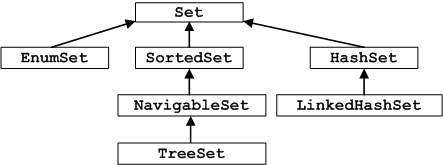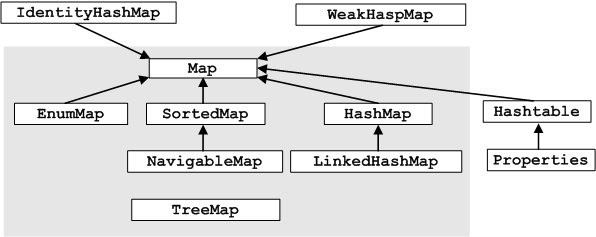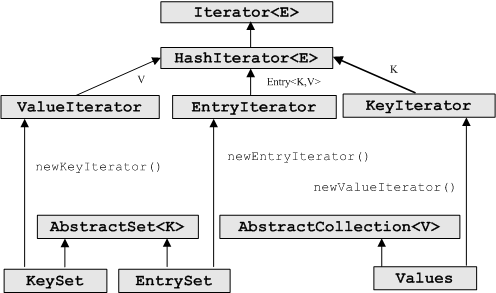# Java 7之集合类型第4篇 - HashMap

		map.put("dbradius","C:/temp/dbradiusx_script.xml");


/*
* 保证脚本可用
*/
public boolean isUsable(){
Iterator<String> iterator = map.keySet().iterator();
while(iterator.hasNext()){
String key = iterator.next();
File f = new File(map.get(key));
if(!f.exists()){
MyTools.getLogger().error(" path " +map.get(key)+ " is unavaible!");
map.remove(key);
}
}
return map.size()>0?true:false;
}

Exception in thread "main" java.util.ConcurrentModificationException
at java.util.HashMap$HashIterator.nextEntry(HashMap.java:793) at java.util.HashMap$KeyIterator.next(HashMap.java:828)
at com.network.manager.nmsmanager.db.util.Bootstrap.isUsable(Bootstrap.java:68)
at com.network.manager.nmsmanager.db.util.Bootstrap.main(Bootstrap.java:49)

while(iterator.hasNext()){
String key = iterator.next();
File f = new File(map.get(key));
if(!f.exists()){
MyTools.getLogger().error(" path " +map.get(key)+ " is unavaible!");
iterator.remove();// 只有在调用过next方法后才可调用iterator的remove
}
}
Iterator 支持从源集合中安全地删除对象，只需在 Iterator 上调用 remove() 即可。这样做的好处是可以避免 ConcurrentModifiedException ，这个异常顾名思意：当打开 Iterator 迭代集合时，同时又在对集合进行修改。有些集合不允许在迭代时删除或添加元素，但是调用Iterator  remove() 方法是个安全的做法。

1. 将Map转换为List类型
2. 通过Entry 遍历Map
3. 通过Key来对Map排序
4. 对value对Map进行排序
5. 初始化一个static 的常量Map
6. HashMap, TreeMap, and Hashtable之间的不同
7. Map中的反向查询
8. 对Map的复制
9. 创建一个空的Map

Map<String, String[]> paraMap = new HashMap<String, String[]>();
for( Entry<String, String[]> entry : paraMap.entrySet() )
{
String appFieldDefId = entry.getKey();
String[] values = entry.getValue();
}

HashMap 的底层由一个散列表来实现，存储的内容是键值对(key-value)，且键值不能重复，最多允许有一个null值。

1、Map与Set的关系

Set集合的特点是不能存储重复元素，不能保持元素插入时的顺序，且key值最多允许有一个null值。Set 集合框架图Map集合框架图

2、Map与Set的关系

Map接口中定义的部分重要方法如下：

public interface Map<K,V> {

boolean containsKey(Object key);
boolean containsValue(Object value);

V get(Object key);

// Modification Operations
V put(K key, V value);
V remove(Object key);

// Bulk Operations
void putAll(Map<? extends K, ? extends V> m);
void clear();

// Views
Set<K> keySet();                 // 由于Map集合的key不能重复，key之间无顺序，所以Map集合中的所有key就可以组成一个Set集合
Collection<V> values();          // 获取所有的values集合
Set<Map.Entry<K, V>> entrySet(); // 获取所有的key-value键值对，由Map.Entry<K,V>来表示

interface Entry<K,V> {           // 表示key-value对实体
K getKey();
V getValue();
V setValue(V value);
// 省略部分常见方法
}
// 省略部分常见的方法
}

1. values() 方法 通过调用这个方法就可以返回Map集合中所有的value值；
2. keySet()方法 调用后可以得到所有Map中的 key值，这些值可以组成一个Set集合
3. entrySet()方法   得到所有的Map中key-value键值对，以Set集合的形式存储。将key看作Set中的元素，value当作 key的附属即可。

3、Map中定义的变量及构造函数

public class HashMap<K,V>  extends AbstractMap<K,V>   implements Map<K,V>, Cloneable, Serializable{

static final int DEFAULT_INITIAL_CAPACITY = 16; // 默认的初始化容量大小，必须是2的平方
static final int MAXIMUM_CAPACITY = 1 << 30;    // 最大的容量

static final float DEFAULT_LOAD_FACTOR = 0.75f; // 指定负载因子

transient Entry[] table;   // 存储key-value对
transient int size;        // 存储的实际key-value对数量
int threshold;             // 所能容纳的key-value对极限
final float loadFactor;    // 负载因子
transient int modCount;    // 记录修改内容的次数
}

1. table数组用来存储key-value对，在调整数组的大小时值肯定是2的平方。

HashMap提供了几个构造函数，如下：

  public HashMap(int initialCapacity, float loadFactor) {
if (initialCapacity < 0)
throw new IllegalArgumentException("Illegal initial capacity: " + initialCapacity);
if (initialCapacity > MAXIMUM_CAPACITY)
initialCapacity = MAXIMUM_CAPACITY;
throw new IllegalArgumentException("Illegal load factor: " + loadFactor);
// Find a power of 2 >= initialCapacity
int capacity = 1;
while (capacity < initialCapacity)        // 计算出大于initialCapacity的最小的2的n次方值
capacity <<= 1;
threshold = (int)(capacity * loadFactor); // 设置容量极限
table = new Entry[capacity];              // 将刚计算出的capacity当作Entry数组的大小
init();
}

public HashMap(int initialCapacity) {
}

public HashMap() {
threshold = (int)(DEFAULT_INITIAL_CAPACITY * DEFAULT_LOAD_FACTOR);
table = new Entry[DEFAULT_INITIAL_CAPACITY];
init();
}

public HashMap(Map<? extends K, ? extends V> m) {
this(Math.max((int) (m.size() / DEFAULT_LOAD_FACTOR) + 1,DEFAULT_INITIAL_CAPACITY), DEFAULT_LOAD_FACTOR);
putAllForCreate(m);
}

4、key-value存储实体 - Entry

private static class Entry<K,V> implements Map.Entry<K,V> { // 实现Map接口中定义的Entry接口
int hash;
K key;
V value;
Entry<K,V> next;
protected Entry(int hash, K key, V value, Entry<K,V> next) {
this.hash = hash;
this.key = key;
this.value = value;
this.next = next;
}
protected Object clone() {
return new Entry<>(hash, key, value,(next==null ? null : (Entry<K,V>) next.clone()));
}
public K getKey() {   // 获取key值
return key;
}
public V getValue() { // 获取value值
return value;
}
public V setValue(V value) { // 为value设置新的值
if (value == null)
throw new NullPointerException();
V oldValue = this.value;
this.value = value;
return oldValue;
}
public boolean equals(Object o) { // 通过比较key和value值来确定两个Entry是否相等
if (!(o instanceof Map.Entry))
return false;
Map.Entry e = (Map.Entry)o;
return (key==null ? e.getKey()==null : key.equals(e.getKey())) &&
(value==null ? e.getValue()==null : value.equals(e.getValue()));
}
public int hashCode() {
return hash ^ (value==null ? 0 : value.hashCode());
}
public String toString() {
return key.toString()+"="+value.toString();
}
}

了解了key-value存储的基本结构后，就可以考虑如何存储的问题了。HashMap顾名思义就是使用哈希表来存储的，哈希表为解决冲突，采用了开放地址法和链地址法来解决问题。Java中HashMap采用了链地址法。链地址法，简单来说，就是数组加链表的结合。在每个数组元素上都一个链表结构，当数据被hash后，得到数组下标，把数据放在对应下标元素的链表上。

当程序试图将多个 key-value 放入 HashMap 中时，以如下代码片段为例：

HashMap<String , Double> map = new HashMap<String , Double>();
map.put("语文" , 80.0);
map.put("数学" , 89.0);
map.put("英语" , 78.2); 
HashMap 采用一种所谓的“Hash 算法”来决定每个元素的存储位置。

当程序执行 map.put("语文" , 80.0); 时，系统将调用"语文"的 hashCode() 方法得到其 hashCode 值——每个 Java 对象都有 hashCode() 方法，都可通过该方法获得它的 hashCode 值。得到这个对象的 hashCode 值之后，系统会根据该 hashCode 值来决定该元素的存储位置。

 Map<String[], String> paraMap = new HashMap<String[], String>();

5、添加元素

HashMap 类的 put(K key , V value) 方法的源代码：

/*
*  当向HashMap中添加mapping时，由key的hashCode值决定Entry对象的存储位置，当两个 key的hashCode相同时,
*  通过equals()方法比较，返回false产生Entry链，true时采用覆盖行为
*/
public V put(K key, V value) {
if (key == null)
return putForNullKey(value);
int hash = hash(key.hashCode());
int i = indexFor(hash, table.length);
for (Entry<K,V> e = table[i]; e != null; e = e.next) {
Object k;
/*
*  如果hash值相同且key值的索引或内容相同，则采取覆盖行为，然后返回旧值
*  这样不会对modCount进行加1操作，也就是说这样不认为是结构发生了改变
*/
if (e.hash == hash && ((k = e.key) == key || key.equals(k))) {
V oldValue = e.value;
e.value = value;
e.recordAccess(this);
return oldValue;
}
}
modCount++;
addEntry(hash, key, value, i);
return null;
} 

当key值为空时，调用putForNullKey()方法进行值的添加，如下：

 private V putForNullKey(V value) {
for (Entry<K,V> e = table; e != null; e = e.next) {
if (e.key == null) {
V oldValue = e.value;
e.value = value;
e.recordAccess(this);
return oldValue;
}
}
modCount++;
addEntry(0, null, value, 0);
return null;
}
void recordAccess(HashMap<K,V> m) {   }

当系统决定存储 HashMap 中的 key-value 对时，完全没有考虑 Entry 中的 value，仅仅只是根据 key 来计算并决定每个 Entry 的存储位置。这也说明了前面的结论：我们完全可以把 Map 集合中的 value 当成 key 的附属，当系统决定了 key 的存储位置之后，value 随之保存在那里即可。

        int hash = hash(key.hashCode());       // 计算hash值
int i = indexFor(hash, table.length);  // 计算保存在table数组索引的位置

根据 hashCode() 返回值来计算 Hash 值的方法，并且会调用 indexFor() 方法来计算该对象应该保存在 table 数组的哪个索引，源代码如下：

    static int hash(int h) {
h ^= (h >>> 20) ^ (h >>> 12);
return h ^ (h >>> 7) ^ (h >>> 4);
}
static int indexFor(int h, int length) {
return h & (length-1);
}

• length是table数组的长度，所以数组的长度总是 2 的 n 次方，通过h&(length-1)计算后，保证计算得到的索引值位于 table 数组的索引之内，计算的方法并不总是这样的，好的计算方法应该还会让索引值尽量平均分布到数组中，以减少冲突。
• hash()方法，目前还没有理解为什么要经过这样的计算

当调用put()方法向 HashMap 中添加 key-value 对，由其 key 的 hashCode() 返回值决定该 key-value 对（就是Entry 对象）的存储位置。当两个 Entry 对象的 key 的 hashCode() 返回值相同时，将由 key 通过 eqauls() 比较值决定是采用覆盖行为（返回 true），还是产生 Entry 链（返回 false），而且新添加的 Entry 位于 Entry 链的头部，这是通过调用addEntry()方法来完成的，源码如下：

void addEntry(int hash, K key, V value, int bucketIndex){
Entry<K,V> e = table[bucketIndex];                        // 获取指定 bucketIndex 索引处的 Entry
table[bucketIndex] = new Entry<K,V>(hash, key, value, e); // 将新创建的 Entry 放入 bucketIndex 索引处，并让新的 Entry 指向原来的 Entry
// 如果 Map 中的 key-value 对的数量超过了极限
if (size++ >= threshold)
resize(2 * table.length); 	                     //  把 table 对象的长度扩充到 2 倍

} 

将新添加的 Entry 对象放入 table数组的 bucketIndex 索引处。如果 bucketIndex 索引处已经有了一个 Entry 对象，那新添加的 Entry 对象指向原有的 Entry 对象（产生一个 Entry 链），如果 bucketIndex 索引处没有 Entry 对象， e 变量是 null，也就是新放入的 Entry 对象指向 null，也就是没有产生 Entry 链。

5、获取元素

get()方法的源代码如下：

public V get(Object key) {
if (key == null)
return getForNullKey();
int hash = hash(key.hashCode());
// 搜索该Entry链的下一个Entry，有多个Entry链时必须顺序遍历，降低了索引的速度
// 如果Entry链过长，说明发生“Hash”冲突比较频繁，需要采用新的算法或增大空间
for (Entry<K,V> e = table[indexFor(hash, table.length)]; e != null; e = e.next) {
Object k;
if (e.hash == hash && ((k = e.key) == key || key.equals(k)))
return e.value;
}
return null;
}

当 HashMap 的每个 bucket 里存储的 Entry 只是单个 Entry 时的 HashMap 具有最好的性能：当程序通过 key 取出对应 value 时，只要先计算出该 key 的 hashCode() 返回值，在根据该 hashCode 返回值找出该 key 在 table 数组中的索引，然后循环遍历查找 hash值相同,key值相同的value。

key为空值时调用getForNullKey()方法，源代码如下：

 private V getForNullKey() {
for (Entry<K,V> e = table; e != null; e = e.next) {
if (e.key == null)
return e.value;
}
return null;
}

key为null的Entry只可能存在于索引值为0处，遍历这个链即可。

6、获取key集合、values集合和Entry集合

transient volatile Set<K>                keySet = null;
transient volatile Collection<V>     values = null;

    private transient Set<Map.Entry<K,V>> entrySet = null;

public Set<K> keySet() {               // 所有的HashMap的key值放入到Set集合
Set<K> ks = keySet;
return (ks != null ? ks : (keySet = new KeySet()));
}
public Collection<V> values() {        // 所有的HashMap的value值放入到Collection集合
Collection<V> vs = values;
return (vs != null ? vs : (values = new Values()));
}
public Set<Map.Entry<K,V>> entrySet() { // 将Entry放到Set集合中
return entrySet0();
}

private Set<Map.Entry<K,V>> entrySet0() {
Set<Map.Entry<K,V>> es = entrySet;
return es != null ? es : (entrySet = new EntrySet());
}private abstract class HashIterator<E> implements Iterator<E> {
Entry<K,V> next;        // next entry to return
int expectedModCount;   // For fast-fail
int index;              // current slot
Entry<K,V> current;     // current entry

HashIterator() {
expectedModCount = modCount;
if (size > 0) { // advance to first entry
Entry[] t = table;
while (index < t.length && (next = t[index++]) == null); // 将index指向第一个table不为null的位置
}
}
public final boolean hasNext() {
return next != null;
}
/*
* 这个方法是final类型的，不可以被子类覆盖，只有包访问权限，所以应用程序不可以调用
* 查找下一个Entry，下一个Entry可能与前一个Entry在不同的链表上。关于为什么这样定义还不是很清楚
*/
final Entry<K,V> nextEntry() {
if (modCount != expectedModCount)
throw new ConcurrentModificationException();
Entry<K,V> e = next;
if (e == null)
throw new NoSuchElementException();
if ((next = e.next) == null) {   // 如果遍历完一个Entry链，则继续查找下一个Entry链的索引
Entry[] t = table;
while (index < t.length && (next = t[index++]) == null);
}
current = e;
return e;
}
public void remove() {
if (current == null)
throw new IllegalStateException();
if (modCount != expectedModCount)
throw new ConcurrentModificationException();
Object k = current.key;
current = null;
HashMap.this.removeEntryForKey(k);
expectedModCount = modCount;
}

}

    private final class EntrySet extends AbstractSet<Map.Entry<K,V>> {
public Iterator<Map.Entry<K,V>> iterator() {
return newEntryIterator();
}
public boolean contains(Object o) {
if (!(o instanceof Map.Entry))
return false;
Map.Entry<K,V> e = (Map.Entry<K,V>) o;
Entry<K,V> candidate = getEntry(e.getKey());
return candidate != null && candidate.equals(e);
}
public boolean remove(Object o) {
return removeMapping(o) != null;
}
public int size() {
return size;
}
public void clear() {
HashMap.this.clear();
}
}


 Iterator<Map.Entry<K,V>> newEntryIterator()   {
return new EntryIterator();
}
 private final class EntryIterator extends HashIterator<Map.Entry<K,V>> {
public Map.Entry<K,V> next() {
return nextEntry();
}
}

HashMap map=new HashMap();
map.put("a", "value1");
map.put("b", "value2");
map.put("c", "value3");

Set<Map.Entry<String,String>> s=map.entrySet();
Iterator iter=s.iterator();
while(iter.hasNext()){
System.out.println(iter.next());
}

b=value2
c=value3
a=value1